May 19, 2019 · 8 min read

# Generative Art: Triangles With p5.js

After going through a bit of history on using computers to make art, my second class on p5.js had us attempt to be inspired by geometric artwork and write code to make something cool.

In about an hour and a half of coding, I ended up with this — randomly generated triangles in all kinds of colors.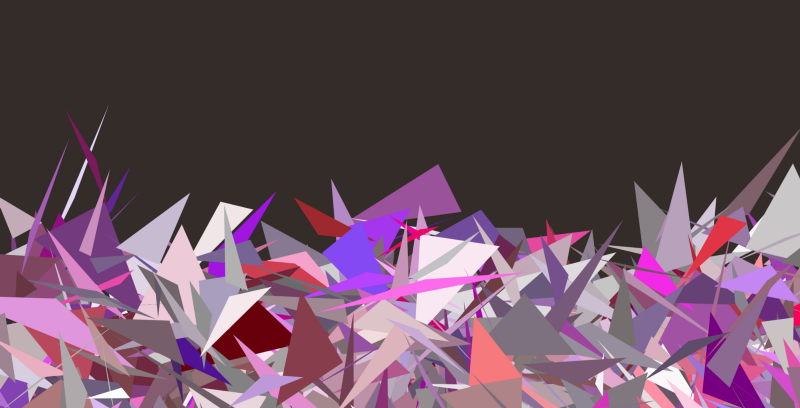We were tasked to continue working on it until we were happy with it — play around with shape, size and color.

I spun some things around in my head during a long commute — I’m determined to get all these triangles to be all connected and not overlap. It was something we discussed before starting the project but we deemed it too difficult to do consider we’re only 3 hours into learning p5. We looked at a library that accomplished it, but I needed to come up with my own solution.

I have a difficult time drawing while I’m driving so as soon as I managed to stop I sketched this out:What if, instead of Random coordinates to create the triangles, I could

• setup a grid on the canvas
• randomly place the points in that grid
• store the coordinates in an array
• then manage to systematically go through and start using those points to create the new triangles

In my head it seems like it could work — getting rid of the randomness of the triangle generation.

Let’s see how far I get into this code before I realize how dumb I was to think this would work.

## Setting up the grid

Just so I can see what my brain is thinking, I’m physically putting the grid onto the canvas:

``````function setup() {
createCanvas( windowWidth, windowHeight )
colorMode( HSB )
noLoop()
noStroke()
}
function draw() {
background( 20, 20, 20)
// Setup a grid so i can figure out the uppper and lower bounds
// of where the points should go
var columns = 5
var rows = 5
var columnWidth = ( windowWidth / columns )
var columnHeight = ( windowHeight / rows )
fill( 30, 30, 30)
stroke( 90, 90, 90 )
rect( 0, 0, columnWidth, columnHeight )
// Create a row
for ( i = 0; i < rows; i++ ){
// Create a column
for ( j = 0; j < columns; j++ ){
rect( j * columnWidth, i * columnHeight, columnWidth, columnHeight )
}
}
}
``````

Which ended up looking like this. I’m off to an okay start.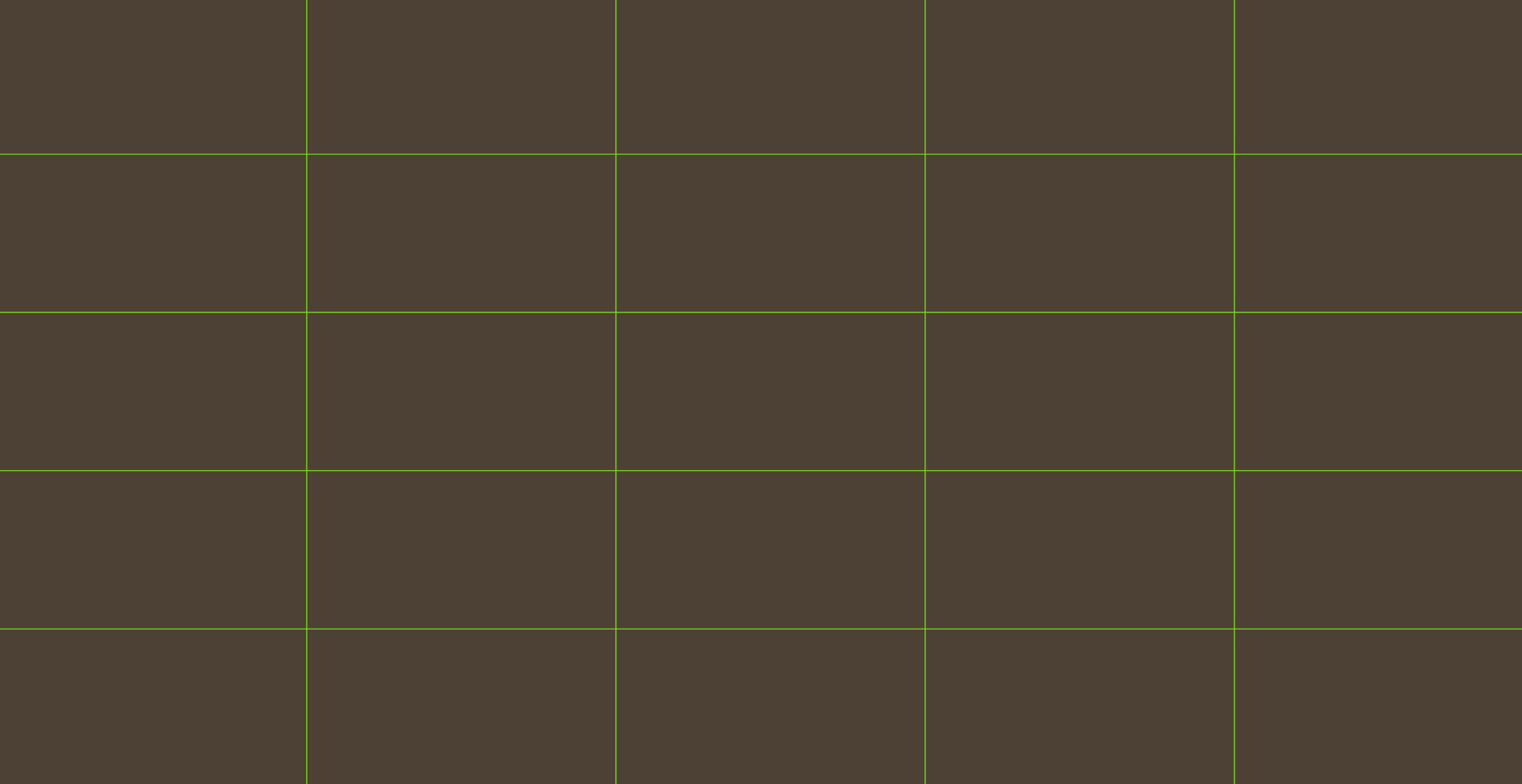## Generating the random points

I’m going to generate random points inside each grid using the upper and lower bounds based on the column width and height and use small circles to visualize where the points are being created.

So just in side the column loop:

``````for ( j = 0; j < columns + 1 ; j++ ){
rect( j * columnWidth, i * columnHeight, columnWidth, columnHeight )
//create my point
var pointPositionX = random( j * columnWidth - columnWidth, j * columnWidth )
var pointPositionY = random( i * columnHeight - columnHeight, i * columnHeight )
circle( pointPositionX, pointPositionY, 10 )
}
``````

and I get some points:## Storing the coordinates in arrays

I need to keep all these coordinates so that I can go back and create the triangles.

``````for ( i = 0; i < rows + 1; i++ ){
var columnData = []
// Create a column
for ( j = 0; j < columns + 1 ; j++ ){
rect( j * columnWidth, i * columnHeight, columnWidth, columnHeight )
//create my point
var pointPositionX = random( j * columnWidth - columnWidth, j * columnWidth )
var pointPositionY = random( i * columnHeight - columnHeight, i * columnHeight )
columnData.push( [pointPositionX, pointPositionY] )
console.log( columnData )
//circle( pointPositionX, pointPositionY, 10 )
}
allCoordinates.push( columnData )
console.log( allCoordinates )
}
``````

It has been a few years since I’ve written some JavaScript but it’s seeming to fit like a glove. This is what was logged to the console. I think I somehow managed to do this nearly right* … except those negative numbers …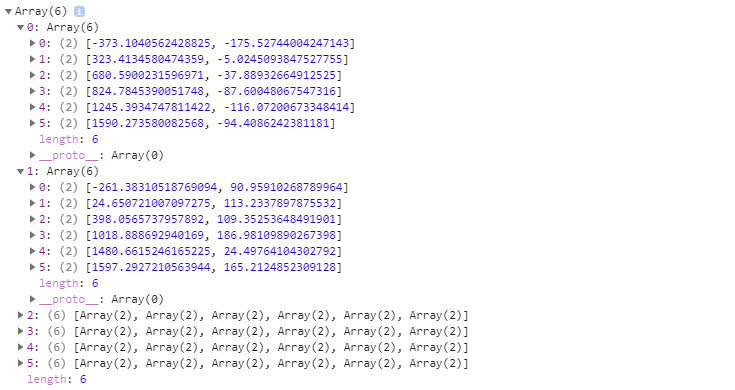## The first triangle

Yeah, so those negative numbers weren’t great. Math is weird sometimes for me — why would I be subtracting from 0? Who knows. I think I thought that i and j were 1’s? Whatever. Fixed it.

Also don’t know why I needed two arrays…I should just push all the coordinates to `allCoordinates[]`.

So once all that was out the way, I wanted to make sure those first points were stored as I expected them and that I could make a triangle from them.

This is what the code ended up being:

``````var allCoordinates = [];
// Create a row
for ( i = 0; i < rows + 1; i++ ){
// Create a column
for ( j = 0; j < columns + 1 ; j++ ){
rect( j * columnWidth, i * columnHeight, columnWidth, columnHeight )
//create my point
var pointPositionX = random( j * columnWidth + columnWidth, j * columnWidth )
var pointPositionY = random( i * columnHeight + columnHeight, i * columnHeight )
allCoordinates.push( [pointPositionX, pointPositionY] )
circle( pointPositionX, pointPositionY, 10 )
console.log(allCoordinates)
}
}
// Create the first triangle
fill( 90, 90, 90 )
var currentPoint = 0
var firstPointX = allCoordinates
var firstPointY = allCoordinates
var secondPointX = allCoordinates
var secondPointY = allCoordinates
var thirdPointX = allCoordinates
var thirdPointY = allCoordinates
triangle( firstPointX,
firstPointY,
secondPointX,
secondPointY,
thirdPointX,
thirdPointY )
``````

and the output: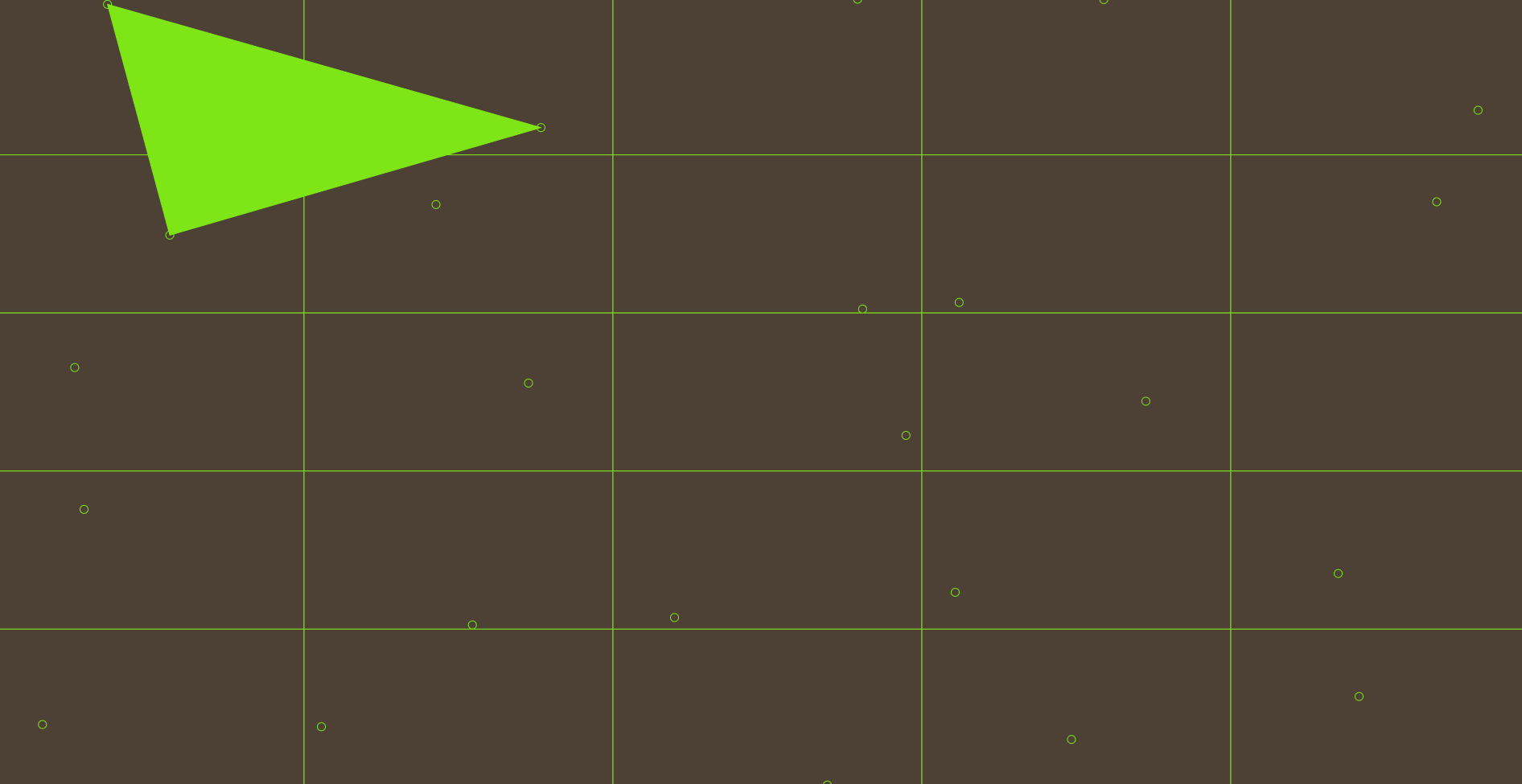Fantastic! Now I gotta loop through and do them all!

## 2 hours of crashing my browser later…

I spent a good bit of time working out how to cycle through each of the points in the array and creating 2 triangles for every point. I messed it up a lot given that I also had to figure out if it was in the first or last row or the last column — it was weird.

I finally got to a point where I was okay with the output and knew that if I just spent another hour on it I could have gotten in nearly perfect…but I was ready to just move on — I’m not trying to overachieve this.

I won’t share that code with you, but here’s how it was shaping up: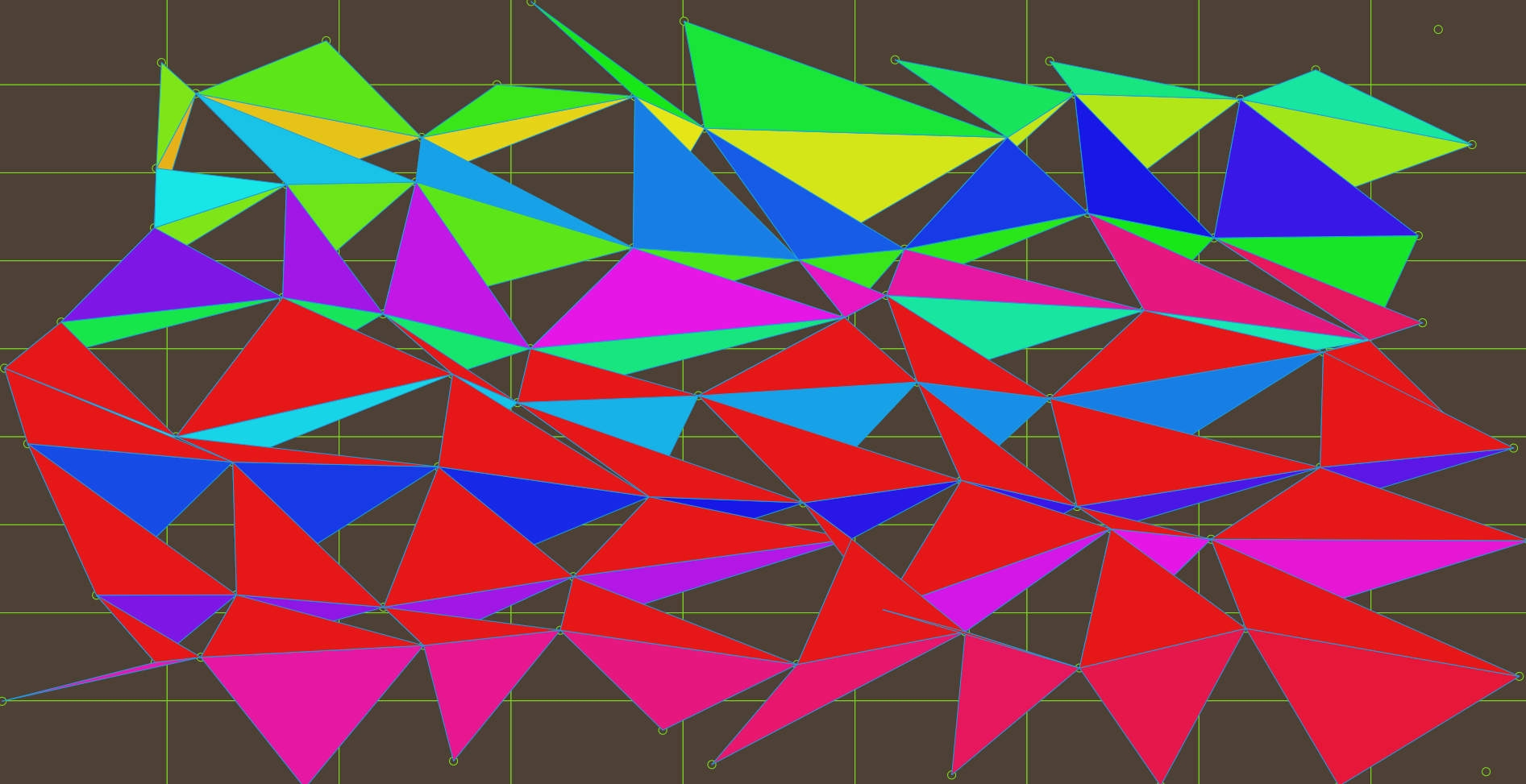## Back to the Art part of this whole thing

With some adjustments of the number of columns and rows, some creative cropping and some actual color choices I came up with a couple iterations before finally enjoying where it was headed. Getting closer…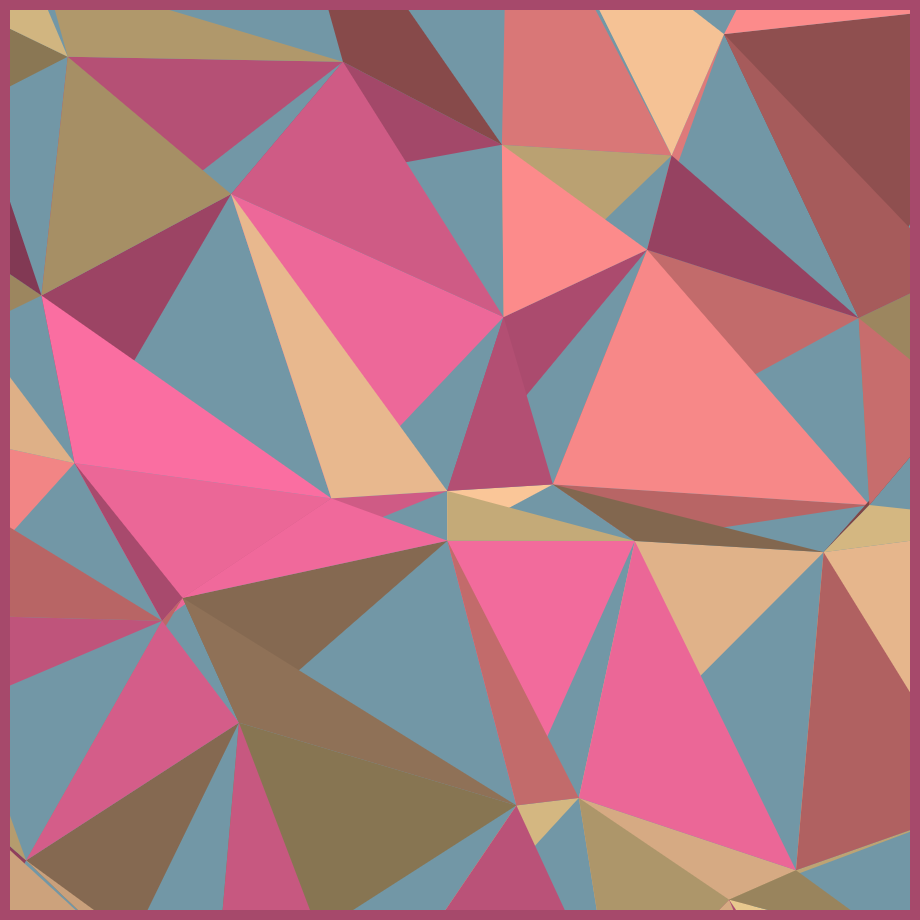## The final render and some pretty sloppy code

Finally, the renders started coming together to a better point — enough where I could say that I was done for now.

This is the final render: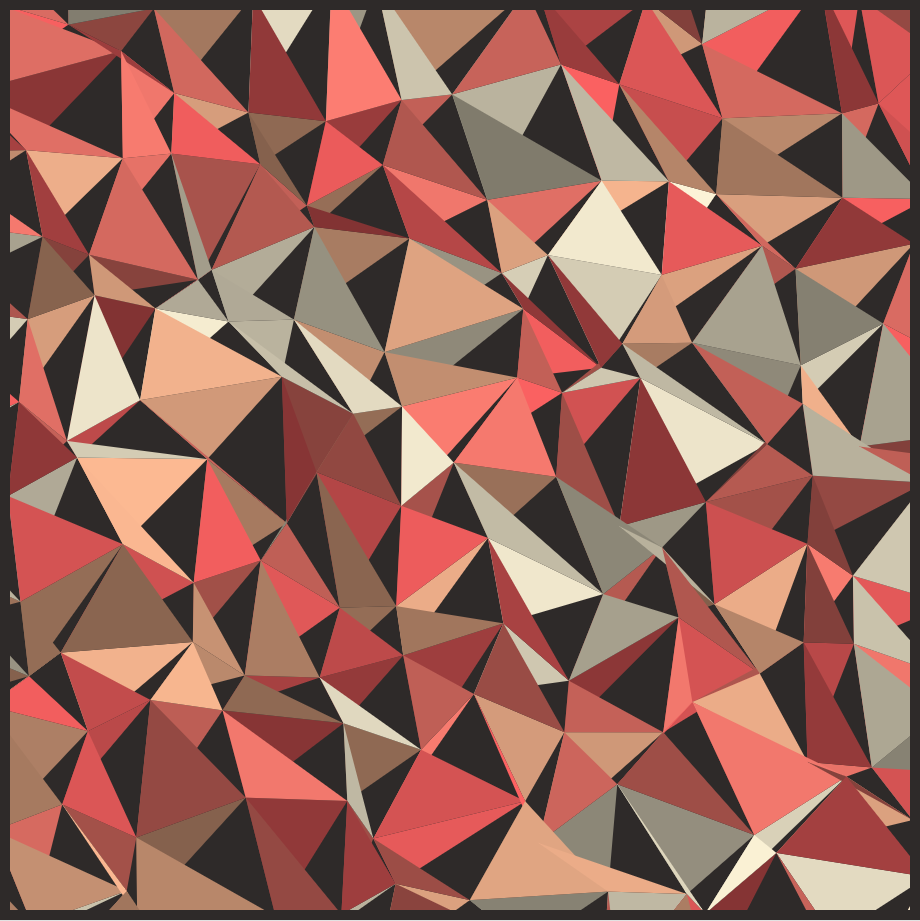And the p5.js code. Sorry, I was ready to call it done today so there’s no cleaning it up. Enjoy it.

``````function setup() {
var canvas = createCanvas( 1600, 1600 )
canvas.parent("canvasArea");
colorMode( HSB )
noLoop()
noStroke()
}

function draw() {
background( 197, 31, 65 )

// Setup a grid so i can figure out the uppper and lower bounds
// of where the points should go
var columns = 20
var rows = 20

var columnWidth = 1600 / columns
var columnHeight = 1600 / rows

// Show the grid
fill( 18, 11, 18 )
//stroke( 90, 90, 90 )
//rect( 0, 0, columnWidth, columnHeight )

var allCoordinates = [];
// Create a row
for ( i = 0; i < rows; i++ ){
// Create a column
for ( j = 0; j < columns ; j++ ){
rect( j * columnWidth, i * columnHeight, columnWidth, columnHeight )
//create my point
var pointPositionX = random( j * columnWidth + columnWidth, j * columnWidth )
var pointPositionY = random( i * columnHeight + columnHeight, i * columnHeight )

allCoordinates.push( [pointPositionX, pointPositionY] )
circle( pointPositionX, pointPositionY, 10 )
}
}
var whichRow = 1
var l = 1
while ( l < allCoordinates.length ){

//firstTriangle
if (whichRow == 1 || whichRow == rows){
//don't do anything on the first and last row (thse need just one triangle)
}
else{
if ( (l + 1) % rows == 0 || l == 0){
console.log("it's the last item, do nothing")
}
else{
//Triangle 1
var colorChoices = [
[45, 15, 75],
[22, 42, 95],
[5, 55, 75],
[0, 61, 95]
]

var randomColorChoice = Math.floor(random(0,4))
fill( colorChoices[randomColorChoice], colorChoices[randomColorChoice], Math.floor(random(50,100)) )
//stroke( 200, 90, 90 )

var currentPoint = l
var nextPoint = l + 1
var bottomPoint = l + rows
var firstPointX = allCoordinates[currentPoint]
var firstPointY = allCoordinates[currentPoint]
var secondPointX = allCoordinates[nextPoint]
var secondPointY = allCoordinates[nextPoint]
var thirdPointX = allCoordinates[bottomPoint]
var thirdPointY = allCoordinates[bottomPoint]
triangle(firstPointX, firstPointY, secondPointX, secondPointY, thirdPointX, thirdPointY)

//Triangle 2
randomColorChoice = Math.floor(random(0,4))
fill( colorChoices[randomColorChoice], colorChoices[randomColorChoice], Math.floor(random(50,100)) )
//stroke( 200, 90, 90 )

var currentPoint = l
var nextPoint = l - rows
var bottomPoint = l + 1
var firstPointX = allCoordinates[currentPoint]
var firstPointY = allCoordinates[currentPoint]
var secondPointX = allCoordinates[nextPoint]
var secondPointY = allCoordinates[nextPoint]
var thirdPointX = allCoordinates[bottomPoint]
var thirdPointY = allCoordinates[bottomPoint]
triangle( firstPointX, firstPointY, secondPointX, secondPointY, thirdPointX, thirdPointY )
}
}
l++
if ( l % rows == 0 ){ whichRow++ }
}
}
``````

## Working demo

Check out the working demo.

## Document Metadata

Raw File With Signature: generative-art-triangles-with-p5js.txt.asc

Hash of Raw File: 7b9d40124636dee6e067ee5dabcaf30fef18dc956bbdcfe67d7707c2e1ae38c1

Ethereum Proof of Existance Transaction: 0xc81faf90051fa5f760e863d0ccd2b4a9f857132b74f4b12457c6ec133a4a8ba0

This text is Creative Commons:Attribution-ShareAlike 4.0 International (CC BY-SA 4.0)